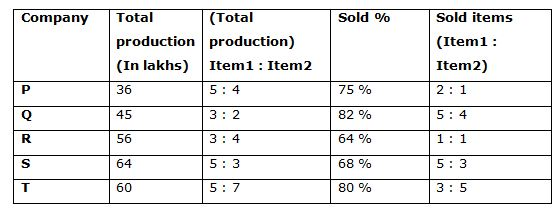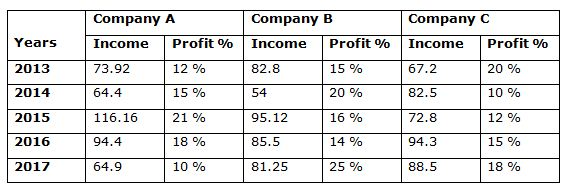# Quantitative Aptitude Questions (Data Interpretation) for SBI Clerk Mains 2018 Day-159

Dear Readers, SBI is conducting Online Examination for the recruitment of clerical cadre To enrich your preparation here we have providing new series of Data Interpretation – Quantitative Aptitude Questions. Candidates those who are appearing in SBI Clerk Mains Exams can practice these Quantitative Aptitude average questions daily and make your preparation effective.

[WpProQuiz 2932]

Click “Start Quiz” to attend these Questions and view Solutions

Directions (Q. 1 – 5) Study the following information carefully and answer the given questions:

Following table shows the total production of 5 different companies and the ratio of production of Item1 and Item2 and the percentage of sold items and the ratio of sold Item1 and Item2 among them.1) Total production of item1 of Company P and R together is approximately what percentage of total production of item2 of Company Q, S and T together?

a) 40 %

b) 80 %

c) 60 %

d) 100 %

e) 50 %

2) Find the difference between the total sold item1 of Company Q and T together to that of total sold item2 of Company P and S together?

a) 1318000

b) 1524000

c) 1636000

d) 1842000

e) None of these

3) Find the average sold item 2 Company Q, R and T together?

a) 23.56 lakhs

b) 21.44 lakhs

c) 25.72 lakhs

d) 24.28 lakhs

e) None of these

4) Find the ratio between the total sold items of Company P and Q together to that of total sold items of Company T?

a) 177: 112

b) 56: 23

c) 324: 217

d) 213: 160

e) None of these

5) Total sold items of Company P is approximately what percentage of total sold items of Company T?

a) 60 %

b) 75 %

c) 90 %

d) 50 %

e) 100 %

Directions (Q. 6 – 10) Study the following information carefully and answer the given questions:

Following table shows the income (In lakhs) and profit % of company A, B and C over the years.Note:

Profit % = [(Income – Expenditure)/Expenditure]*100

6) Total income of Company A in all the given years together is approximately what percentage of total income of Company B in all the given years together?

a) 120 %

b) 95 %

c) 105 %

d) 130 %

e) 75 %

7) Find the ratio between the total expenditure of Company B in the year 2013 and 2016 together to that of total expenditure of Company C in the year 2013, 2015 and 2017 together?

a) 3: 4

b) 4: 7

c) 5: 8

d) 6: 7

e) None of these

8) Find the difference between the total expenditure of Company A in the year 2013 and 2016 together to that of total expenditure of Company C in the year 2014 and 2017 together?

a) 8 lakhs

b) 2 lakhs

c) 6 lakhs

d) 4 lakhs

e) None of these

9) Find the average income of Company C in all the given years together?

a) 7852000

b) 8106000

c) 7634000

d) 8378000

e) None of these

10) Find the total expenditure of Company B in the year 2014, 2015 and 2017 together?

a) 216 lakhs

b) 184 lakhs

c) 192 lakhs

d) 175 lakhs

e) 208 lakhs

Total production of item1 of Company P and R together

= > 36*(5/9) + 56*(3/7)

= > 20 + 24 = 44 lakhs

Total production of item2 of Company Q, S and T together

= > 45*(2/5) + 64*(3/8) + 60*(7/12)

= > 18 + 24 + 35 = 77 lakhs

Required % = (44/77)*100 = 57 %

The total sold item1 of Company Q and T together

= > 45*(82/100)*(5/9) + 60*(80/100)*(3/8)

= > 20.5 + 18 = 38.5 lakhs

The total sold item2 of Company P and S together

= > 36*(75/100)*(1/3) + 64*(68/100)*(3/8)

= > 9 + 16.32 = 25.32 lakhs

Required difference = 38.5 – 25.32 = 13.18 lakhs = 1318000

The total sold item2 Company Q, R and T together

= > 45*(82/100)*(4/9) + 56*(64/100)*(1/2) + 60*(80/100)*(5/8)

= > 16.4 + 17.92 + 30 = 64.32 lakhs

Required average = (64.32/3) = 21.44 lakhs

The total sold items of Company P and Q together

= > 36*(75/100) + 45*(82/100)

= > 27 + 36.9 = 63.9 lakhs

The total sold items of Company T

= > 60*(80/100)

= > 48

Required ratio = 63.9: 48 = 213: 160

Total sold items of Company P

= > 36*(75/100) = 27 lakhs

Total sold items of Company T

= > 60*(80/100) = 48 lakhs

Required % = (27/48)*100 = 57 % = 60 %

Direction (6-10)

Total income of Company A in all the given years together

= > 73.92 + 64.4 + 116.16 + 94.4 + 64.9

= > 413.78 lakhs

Total income of Company B in all the given years together

= > 82.8 + 54 + 95.12 + 85.5 + 81.25

= > 398.67 lakhs

Required % = (413.78/398.67)*100 = 105 %

The total expenditure of Company B in the year 2013 and 2016 together

= > 82.8*(100/115) + 85.5*(100/114)

= > 72 + 75 = 147

The total expenditure of Company C in the year 2013, 2015 and 2017 together

= > 67.2*(100/120) + 72.8*(100/112) + 88.5*(100/118)

= > 56 + 65 + 75 = 196

Required ratio = 147: 196 = 3: 4

The total expenditure of Company A in the year 2013 and 2016 together

= > 73.92*(100/112) + 94.4*(100/118)

= > 66 + 80 = 146 lakhs

The total expenditure of Company C in the year 2014 and 2017 together

= > 82.5*(100/110) + 88.5*(100/118)

= > 75 + 75 = 150 lakhs

Required difference = 150 – 146 = 4 lakhs

The average income of Company C in all the given years together

= > (67.2 + 82.5 + 72.8 + 94.3 + 88.5)/5

= > 405.3/5

= > 81.06 lakhs = 8106000

The total expenditure of Company B in the year 2014, 2015 and 2017 together

= > 54*(100/120) + 95.12*(100/116) + 81.25*(100/125)

= > 45 + 82 + 65

= > 192 lakhs

Daily Practice Test Schedule | Good Luck

 Topic Daily Publishing Time Daily News Papers & Editorials 8.00 AM Current Affairs Quiz 9.00 AM Quantitative Aptitude “20-20” 11.00 AM Vocabulary (Based on The Hindu) 12.00 PM General Awareness “20-20” 1.00 PM English Language “20-20” 2.00 PM Reasoning Puzzles & Seating 4.00 PM Daily Current Affairs Updates 5.00 PM Data Interpretation / Application Sums (Topic Wise) 6.00 PM Reasoning Ability “20-20” 7.00 PM English Language (New Pattern Questions) 8.00 PM